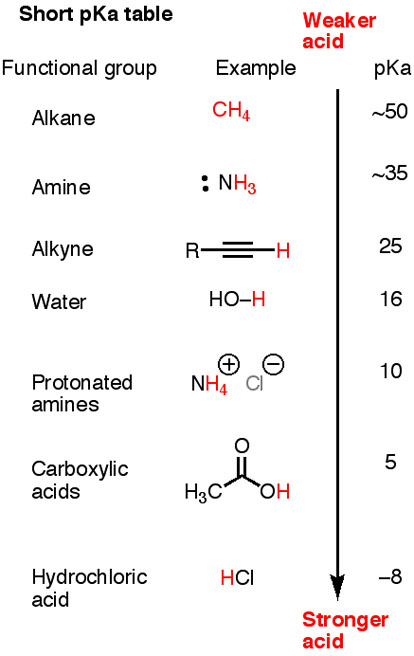# What conjugate base(s) will deprotonate water?

Apr 10, 2014

The conjugate base of any acid with a $p {K}_{\text{a}}$ greater than water will deprotonate water.

#### Explanation:

Here is a short $p {K}_{\text{a}}$ table.Note that $\text{HC≡C-H", "H"_2"N-H}$, and $\text{H"_3"C-H}$ are all weaker acids than water.

The corresponding conjugate bases, $\text{H-C≡C:"^"-", "H"_2"N:"^"-}$, and $\text{H"_3"C:"^"-}$, are strong. They will all deprotonate water.

The conjugate base of methane $\text{H"_3"C:"^"-}$ is strong enough to deprotonate anything below it in the table.

Methyllithium, $\text{CH"_3"Li}$, is one of the strongest bases around.

Acetylide ion ($\text{H-C≡C:"^"-}$) is strong enough to deprotonate only an acid with a $\text{pK"_"a} < 25$.

Acetate ion ($\text{CH"_3"COO"^"-}$) is weaker still, able to deprotonate only an acid with a $p {K}_{a} < 5$.

A more complete table of $p {K}_{\text{a}}$ values will give you many more conjugate bases that will deprotonate water.